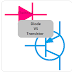The main difference between a diode and a transistor is the diode is a two-terminal device whose main function is to allow the flow of current in one direction only whereas a Transistor is a three-terminal device whose main function is to switch or amplify the electrical or electronic signal.

The other important differences between Diode and Transistor are,
1. The diode is basically a two-layer device such as a PN Junction Diode that has a P-Type Layer and an N-Type Layer. On the other hand, A transistor is basically a three-layer device such as a PNP transistor has two P-type layers and one N-type layer.
2. A diode will show a single VI characteristic but a transistor will show different VI characteristics for different control or biasing values.
3. The diode allows the flow of current in one direction only whereas the transistor(such as BJT) may allow the flow of current in both directions.
4. The terminals of a Diode are named as Anode and Cathode whereas the terminals of a transistor are named as - Collector, Base, Emitter or Gate, Drain, Source.
5. A diode cannot vary the magnitude of the voltage or current flowing through it but a transistor can increase the magnitude of the input signal with the help of a reference signal.

## How does a Diode work?

There are different types of the diode and they have different working principles. Anyway, we can discuss about a normal PN Junction Diode. As we know that the diode is a two-terminal device. So it is to be connected in forwarding bias(if you want to allow the current) or is to be connected in reverse bias (if you want to block the current).

In forward bias, the diode requires a minimum threshold voltage to start the current flow. In reverse bias, the depletion region becomes wider so the flow of current will be blocked. But remember that a very reverse voltage applied to the diode can turn it into breakdown mode and it will start conducting current. Diodes are mostly used for rectification purposes such as full-wave and half-wave rectifiers. Half wave rectifier is built with a single PN Junction Diode whereas the full-wave rectifier is built with either two PN Junction Diode or four PN Junction Diode. This is also known as a rectifier diode.

## How does a Transistor work?

A transistor is a three-terminal device. So one terminal is used as an input terminal and one terminal is used as an output terminal and the other terminal can be used as a common terminal for both input and output.

For example, in a common emitter amplifier using BJT, the input signal is to be applied across the base and emitter terminal whereas the output can be obtained across the collector and emitter terminal. The transistor will generate a high-magnitude signal just the same as the input signal with the help of a reference or biasing power supply. You can see the application of transistors everywhere in electronic circuits such as amplifiers, logic gates, and switching circuits. Even a transistor is the fundamental component of the digital circuit.

The Main Difference between Diode and TransistorReviewed by Author on April 10, 2022 Rating: 5